# DAV Class 6 Maths Chapter 14 Worksheet 3 Solutions

The DAV Class 6 Maths Book Solutions and DAV Class 6 Maths Chapter 14 Worksheet 3 Solutions of Constructions offer comprehensive answers to textbook questions.

## DAV Class 6 Maths Ch 14 WS 3 Solutions

Question 1.
Draw the following angles using ruler and compasses. Also label them:
(a) 60°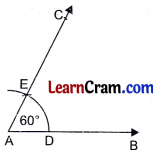Draw a ray AB.
Draw an arc with centre A and any suitable radius intersecting AB at D.
Taking D as centre and radius equal to the radius same as in step 2, draw an arc intersecting the first arc at E.
Join AE and produce to C. Then ∠CAB = 60°

(b) 120°Draw a ray AB.
Taking A as centre and with suitable radius, draw an arc intersecting AB at D.
Draw an arc with centre D and radius equal to first arc intersecting it at E.
Draw one more arc with centre E and the radius equal to first, intersects the previous arc at F. Join AF and produce to C.
Then ∠CAB = 120°.

(c) 90°Draw a ray AB.
Draw an arc with centre A and suitable radius + intersecting it at D.
Draw an arc with centre D and radius equal to first, intersecting the previous arc at E.
Draw another arc with centre E and radius equal to the previous arc intersecting it at F.
Taking E and F as centres and suitable radius, draw to circles intersecting each other at G.
Join AG and produce to C. Then ∠CAB = 90°.

(d) 45°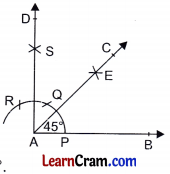Draw a ray AB.
Take A as centre and draw an arc cutting AB at P.
Taking P as centre, mark an arc at Q and then mark another arc at R taking Q as centre.
Draw two arcs taking R and Q as centres with the same radius which cut each other at S.
Join AS and produce to D such that ∠DAB = 90°. Draw AC as the bisector of ∠DAB.
Hence ∠CAB – 45°.

(e) 30°Draw a ray AB.
Taking A as centre draw an arc meeting AB at P. With the same radius taken in step 2 and with centre P, mark an arc Q.
Join AQ and produce to C such that ∠CAB = 60°. Draw AR as the bisector of ∠CAB.
Hence ∠CAR = 30°.

(f) 180°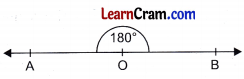Draw a straight line AB.
Take any point 0 on AB.
Hence ∠AOB = 180°.Question 2.
Construct an angle of 30° using compasses and ruler. Now construct an angle of measure 15° using compasses.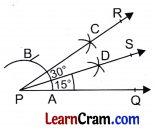Step 1: Draw a ray PQuestion
Step 2: Draw an arc with centre P and suitable radius intersecting it at A.
Step 3: Draw an arc with centre A and radius equal to first, intersecting the previous arc at B.
Step 4: With A and B as centres and suitable radius, draw two arcs intersecting at C.
Step 5: Join PC and produce to R such that ∠RPQ = 30°.
Step 6: Draw PS as the bisector of ∠RPQ with similar method.
Then ∠SPQ = 15°

Question 3.
Construct an angle of measure 22 $$\frac{1}{2}$$° using compasses and ruler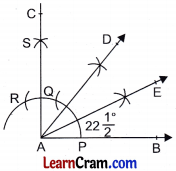Hence ∠EAB = 22$$\frac{1}{2}$$°